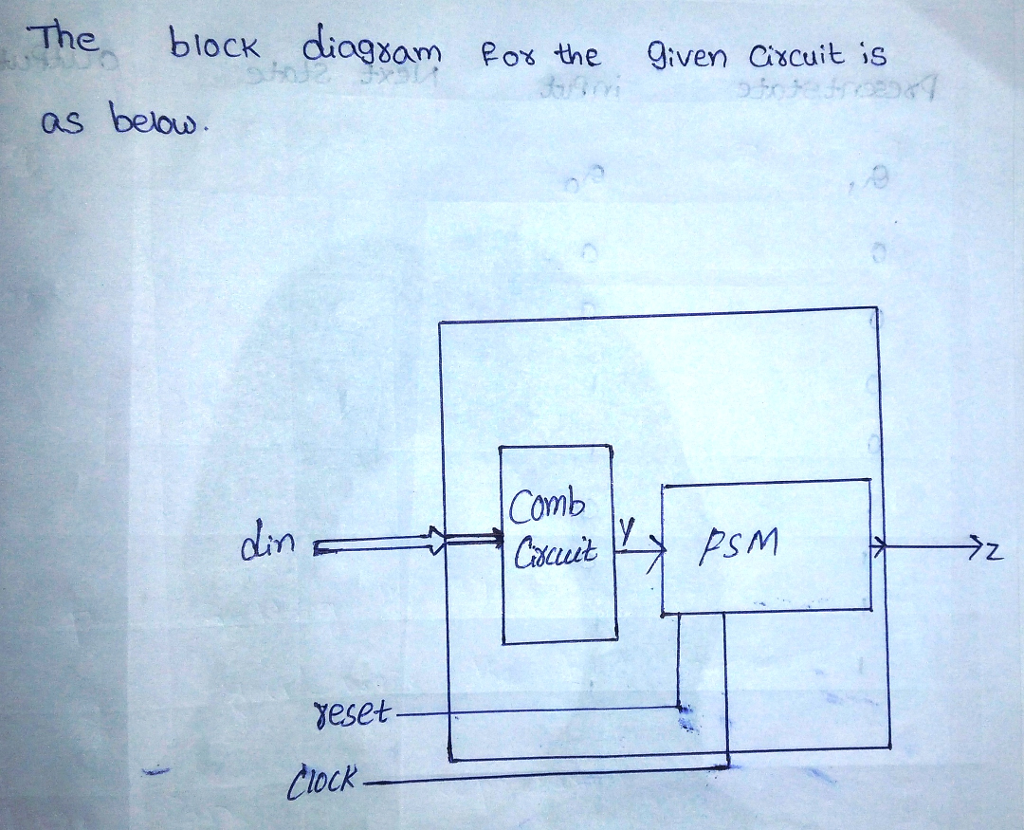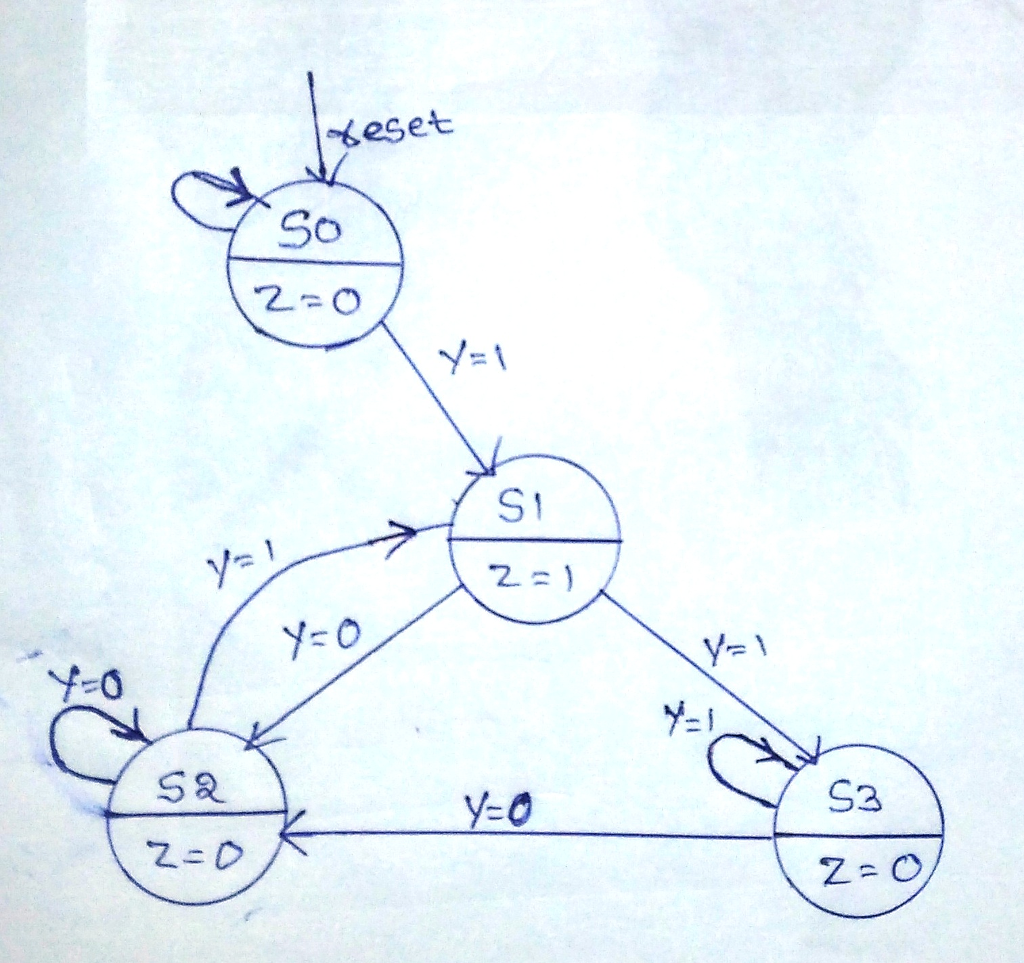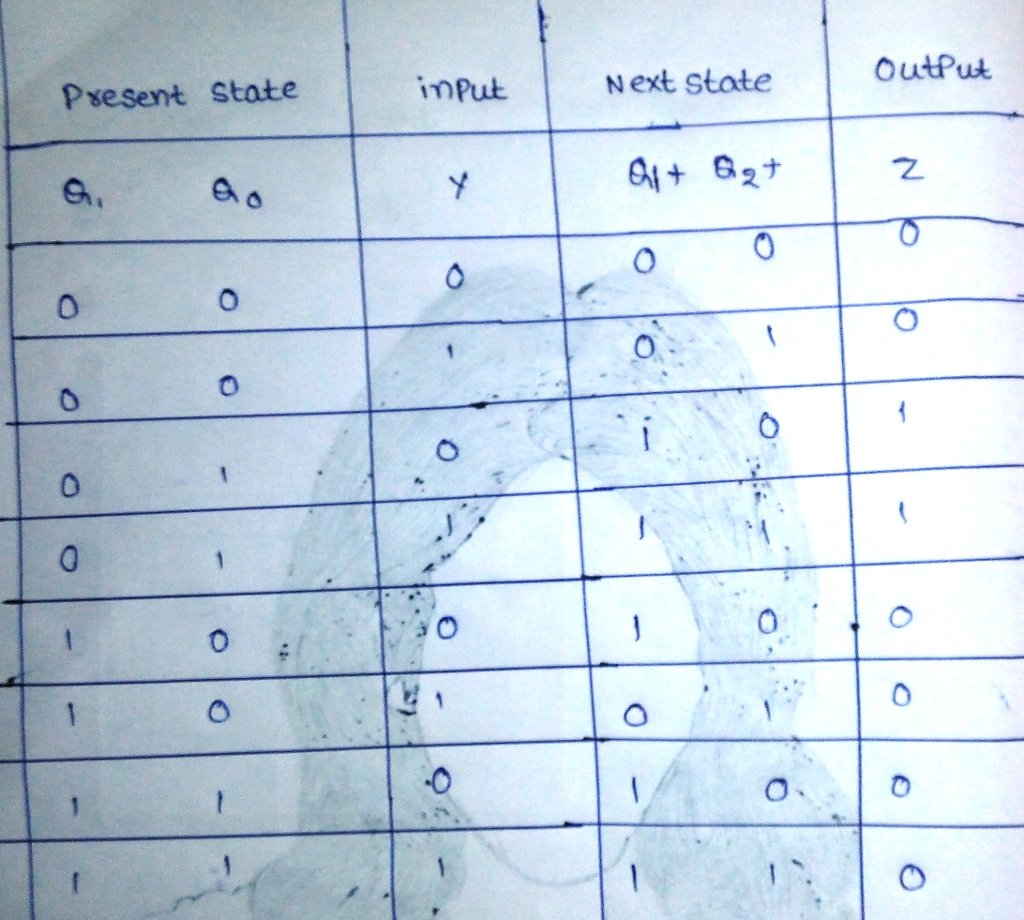# Question & Answer: You are to design a one-shot circuit that has eight inputs and an output. The circuit will generate a single pulse at the positive edge of the…..

You are to design a one-shot circuit that has eight inputs and an output. The circuit will generate a single pulse at the positive edge of the incoming clock signal whenever it receives (AA)16 in its input data lines after this pulse a new pulse will not be generated unless the inputs are returned to any other combination (Other than AA) and again receive (AA)16 in its input lines. Use behavioral form of VHDL design.

Don't use plagiarized sources. Get Your Custom Essay on
Question & Answer: You are to design a one-shot circuit that has eight inputs and an output. The circuit will generate a single pulse at the positive edge of the…..
GET AN ESSAY WRITTEN FOR YOU FROM AS LOW AS \$13/PAGEHere din is the 8 bit input and z is 1-bit output(for pulse). In addition, reset and clock are two more inputs. Since din is 8 bit and we have to detect pattern (AA)16, we will have to give din as input to a combinational circuit whose output is y(1 bit).

y=1 if din=(AA)16 else

y=0

This y is then given as input to the FSM that we have designed.

Thus, FSM has three 1-bit inputs: reset,clock and y and one 1-bit output:z(which is the final output of the circuit). The state diagram for the FSM is as follows:As there are four states, we need two flipflops(output=Q1Q0) for state encoding:
if state=S0 then Q1Q0=00

if state=S1 then Q1Q0=01

if state=S2 then Q1Q0=10

if state=S3 then Q1Q0=11

So, our state transition is as below: